# Sets

I don't want to belong to any club that will accept people like me as a member.

Groucho Marx

In our quest to reason about quantities and relationships, we'd like to handle quantities that are a bit more general than integers (real numbers, for example). We'd also like to handle relationships. To do this we will develop a language that is essentially equivalent to logic, but is a bit more intuitive to use. That language is the language of sets.

### What is a set?

The intuitive definition of a set is just a collection of things. This is, of course, not much of a definition. Many mathematicians in the late nineteenth century actually thought the precise definition of set did not much matter. But the philosopher and mathematician Bertrand Russell, writing in 1901, discovered that we have to be more careful. He considered the following:

Definition. We call a collection ordinary if it does not contain itself. Otherwise we call the collection extraordinary.

Most collections are ordinary, for example the collection of all Bernese mountain dogs is not itself a Bernese mountain dog. The set of all natural numbers is not itself a natural number. But some collections are extraordinary, for example:

• The collection of abstract ideas is an abstract idea.
• The collection of mathematical objects is a mathematical object.
• The collection of all collections is a collection.

Russell therefore asked the question: Is the collection of ordinary collections ordinary? To make this easier, let's write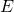for the collection of extraordinary collections,for the collection of ordinary collections, and write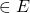and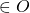for is extraordinary" and is ordinary", respectively. Thus a collection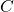satisfies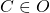exactly when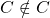and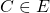exactly when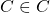.

There are two possibilities. If the collection of ordinary collections is ordinary (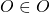), then we know thatmust be ordinary, socannot contain itself, i.e.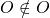. If the collection of ordinary collections is extraordinary, (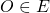), then we know thatmust contain itself, so. But a collection cannot be both ordinary and extraordinary.

So such a collection leads to a paradox--precisely as the sentence this sentence is false did. Russell's paradox shows that we need to be very careful about the words is in".

Definition. A set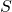is an object for which the question Is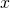in?" has an unambiguous answer. We write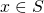(readis an element of") if the answer is yes and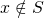if the answer is no.

Another way to phrase this is:is a set exactly whenis an open sentence.

Published in sets.